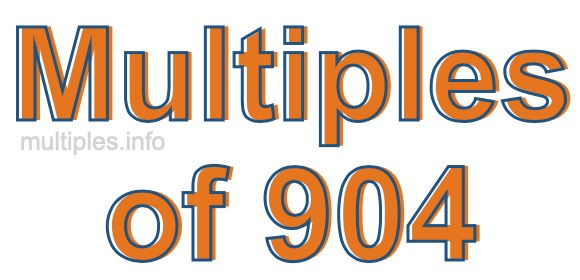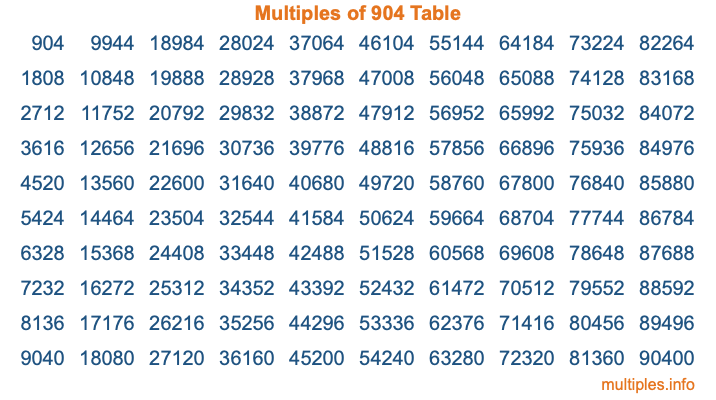Multiples of 904Welcome to the Multiples of 904 page. Here we will first teach you everything you will ever need to know about the multiples of 904, and then give you a study guide summary of everything we taught you to make sure you remember it all. Use this page to look up facts and learn information about the multiples of 904. This page will make you a multiples of nine hundred four expert!

Definition of Multiples of 904
Multiples of 904 are all the numbers that when divided by 904 equal an integer. Each of the multiples of 904 are called a multiple. A multiple of 904 is created by multiplying 904 by an integer.

Therefore, to create a list of multiples of 904, you start with 1 multiplied by 904, then 2 multiplied by 904, then 3 multiplied by 904, and so on for as long as you want. Thus, the list of the first five multiples of 904 is 904, 1808, 2712, 3616, and 4520. To see a larger list of multiples of 904, see the printable image of Multiples of 904 further down on this page. We also have a category where you can choose any nth multiple of 904.

Multiples of 904 Checker
The Multiples of 904 Checker below checks to see if any number of your choice is a multiple of 904. In other words, it checks to see if there is any number (integer) that when multiplied by 904 will equal your number. To do that, we divide your number by 904. If the the quotient is an integer, then your number is a multiple of 904.

Is  a multiple of 904?

Least Common Multiple of 904 and ...
A Least Common Multiple (LCM) is the lowest multiple that two or more numbers have in common. This is also called the smallest common multiple or lowest common multiple and is useful to know when you are adding our subtracting fractions. Enter one or more numbers below (904 is already entered) to find the LCM.

Check out our LCM Calculator if you need more details about the Least Common Multiple or if you need the LCM for different numbers for adding and subtraction fractions.

nth Multiple of 904
As we stated above, 904 is the first multiple of 904, 1808 is the second multiple of 904, 2712 is the third multiple of 904, and so on. Enter a number below to find the nth multiple of 904.

th multiple of 904

Multiples of 904 vs Factors of 904
904 is a multiple of 904 and a factor of 904, but that is where the similarities end. All postive multiples of 904 are 904 or greater than 904. All positive factors of 904 are 904 or less than 904.

Below is the beginning list of multiples of 904 and the factors of 904 so you can compare:

Multiples of 904: 904, 1808, 2712, 3616, 4520, etc.

Factors of 904: 1, 2, 4, 8, 113, 226, 452, 904

As you can see, the multiples of 904 are all the numbers that you can divide by 904 to get a whole number. The factors of 904, on the other hand, are all the whole numbers that you can multiply by another whole number to get 904.

It's also interesting to note that if a number (x) is a factor of 904, then 904 will also be a multiple of that number (x).

Multiples of 904 vs Divisors of 904
The divisors of 904 are all the integers that 904 can be divided by evenly. Below is a list of the divisors of 904.

Divisors of 904: 1, 2, 4, 8, 113, 226, 452, 904

The interesting thing to note here is that if you take any multiple of 904 and divide it by a divisor of 904, you will see that the quotient is an integer.

Multiples of 904 Table
Below is an image of the first 100 multiples of 904 in a table. The table is in chronological order, column by column. The first column has the first ten multiples of 904, the second column has the next ten multiples of 904, and so on.The Multiples of 904 Table is also referred to as the 904 Times Table or Times Table of 904. You are welcome to print out our table for your studies.

Negative Multiples of 904
Although not often discussed or needed in math, it is worth mentioning that you can make a list of negative multiples of 904 by multiplying 904 by -1, then by -2, then by -3, and so on, to get the following list of negative multiples of 904:

-904, -1808, -2712, -3616, -4520, etc.

Multiples of 904 Summary
Below is a summary of important Multiples of 904 facts that we have discussed on this page. To retain the knowledge on this page, we recommend that you read through the summary and explain to yourself or a study partner why they hold true.

There are an infinite number of multiples of 904.

A multiple of 904 divided by 904 will equal a whole number.

904 divided by a factor of 904 equals a divisor of 904.

The nth multiple of 904 is n times 904.

The largest factor of 904 is equal to the first positive multiple of 904.

904 is a multiple of every factor of 904.

904 is a multiple of 904.

A multiple of 904 divided by a divisor of 904 equals an integer.

904 divided by a divisor of 904 equals a factor of 904.

Any integer times 904 will equal a multiple of 904.

Multiples of a Number
Here you can get the multiples of another number, all with the same attention to detail as we did for multiples of 904 on this page.

Multiples of
Multiples of 905
Did you find our page about multiples of nine hundred four educational? Do you want more knowledge? Check out the multiples of the next number on our list!